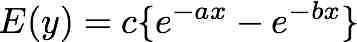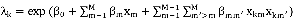Part A. Minimax Optimal Design
Reference.
• R.-B. Chen, S.-P. Chang, W. Wang, and W. K. Wong. (2011) "Optimal Experimental Designs via Particle Swarm Optimization Methods." Technique report. [download]
• Wong, W. K. (1995). On the equivalence of D and G-optimal Designs in Heteroscedastic Models. Statistics & Probability Letters, Vol. 25, 317-321. [link]
• Dette, H. and Wong, W. K. (1999). E-optimal Designs for the Michaelis-Menten Model. Statistics & Probability Letters, Vol. 44, 405-408. [link]

Part B. Mixture Experiment
Reference.
• W. Wang, R.-B. Chen, C.-C. Huang, and W. K. Wong. (2012) "Particle Swarm Optimization Techniques for Finding Optimal Mixture Designs." Technique report. [download]

Part C. Locally D- and Ds-optimal Designs for Logistic Models
Reference.
• J. Qiu, R.-B. Chen, W. Wang, W. K. Wong (2012) .“Using Animal Instincts to Design Efficient Studies,” Technique report. [download]
• Forniusa, E. F. and Nyquista, H. (2009) Using the Canonical Design Space to Obtain c-optimal Designs for the Quadratic Logistic Model. Communications in Statistics - Theory and Methods, Volume 39, 144-157

Part D. Locally designs for compartmental model
The model is.
Reference.
• J. Qiu, R.-B. Chen, W. Wang, W. K. Wong (2012) .“Using Animal Instincts to Design Efficient Studies,” Technique report. [download]
• Atkinson, A. C., Donev, A. N. and Tobias, R. D. (2007). Optimum Experimental Designs, with SAS. Oxford Statistical Science series, Vol. 34. 261-264

Part E. Locally D-optimal designs for 4-parameter Hill model
Reference.
• J. Qiu, R.-B. Chen, W. Wang, W. K. Wong (2012) .“Using Animal Instincts to Design Efficient Studies,” Technique report. [download]
• Leonid A., Khinkis, L. A., Levasseur, L. Faessel, H. and Greco, W. R. (2003). Optimal Design for Estimating Parameters of the 4-parameter Hill Model. Nonlinearity in Biology, Toxicology and Medicine, Vol.1 #3, 363-377.

Part F. Locally D-optimal design for the double-exponential regrowth model
Reference.
• J. Qiu, R.-B. Chen, W. Wang, W. K. Wong (2012) .“Using Animal Instincts to Design Efficient Studies,” Technique report. [download]
• Li G. and Balakrishnan N. (2011) Optimal designs for tumor regrowth models. J. Statist. Plann. Inf., 141, 644-654.

Part G. Two-parameter exponential model with type I right censored data
The probability density function and the corresponding survival function are⁡⁡⁡⁡, where t is the observed value and x is the experimental condition for the observed data.
Reference.
• J. Qiu, R.-B. Chen, W. Wang, W. K. Wong (2012) .“Using Animal Instincts to Design Efficient Studies,” Technique report. [download]
• Konstantinou, M., Biedermann, S. and Kimber, A. (2011). Optimal designs for two-parameter nonlinear models with application to survival models. Southampton Statistical Research Institute, University of Southampton.

Part H. Multivariate exponential and Poisson regression models
For the kth dose combination, xk= (xk1, …, xkM)’, the mean of this M-variable model is assumed to be.
Reference.

Disclaimer of Liability
These programs are provided here for evaluating the capability of particle swarm optimization for finding optimal designs. The codes should be used as they are and without any warranty.